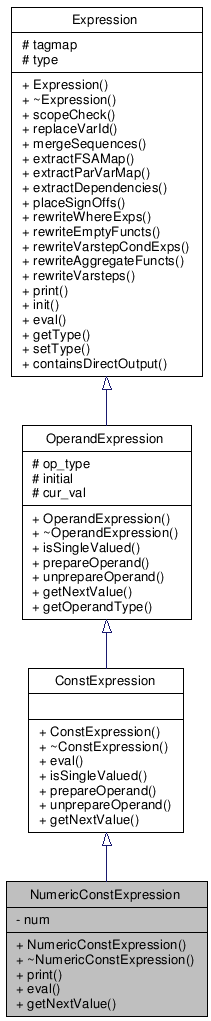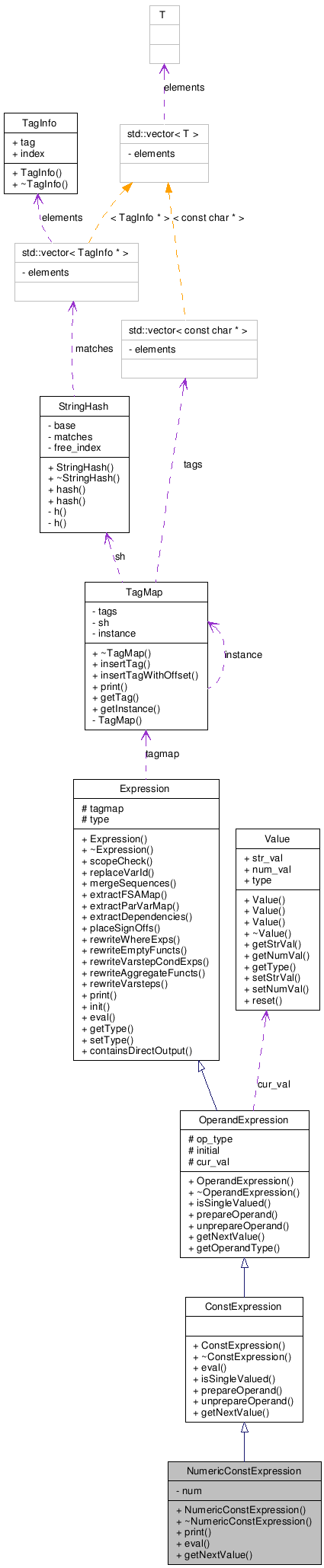# NumericConstExpression Class Reference

Represents an XQuery numeric value. More...

`#include <numericconstexpression.h>`

Inheritance diagram for NumericConstExpression:[legend]
Collaboration diagram for NumericConstExpression:[legend]

List of all members.

## Public Member Functions

NumericConstExpression (const char *_num)
Constructor.
virtual ~NumericConstExpression ()
void print (OutputStream &dos) const
void eval (OutputStream &eos, Environment *env, unsigned modus)
virtual ValuegetNextValue ()

## Private Attributes

long double num
The entered numeric value.

## Detailed Description

Represents an XQuery numeric value.

Represents an XQuery numeric value, e.g. `3`, which takes therefore a numeric value as argument and returns this (numeric) value.

Version:
2.1

Definition at line 49 of file numericconstexpression.h.

## Constructor & Destructor Documentation

 NumericConstExpression::NumericConstExpression ( const char * _num )

Constructor.

Constructor - creating object for a string (numeric value), which will be casted to a numeric value.

Parameters:
 [in] _num Pointer to a char object.

Definition at line 38 of file numericconstexpression.cpp.

 NumericConstExpression::~NumericConstExpression ( ) ` [virtual]`

Destructor.

Definition at line 43 of file numericconstexpression.cpp.

## Member Function Documentation

 void NumericConstExpression::eval ( OutputStream & eos, Environment * env, unsigned modus ) ` [virtual]`

Evaluates the expression in the given environment.

Evaluates the expression in the given environment. The evaluation takes place in the environment that is passed to this method. In addition, the parameter modus is one of the following: EVAL_QUERY, EVAL_QUERY_SILENT or EVAL_SIGNOFF (see also the corresponding defines for more information). This method implements the XQuery semantics for the standard expression types, but also the GCX specific extensions like signOff-statements.

Parameters:
 [in] eos Pointer to a OutputStream object. [in] env Pointer to an Environment object. [in] modus The query evaluation mode.
Exceptions:
 RuntimeException If illegal evaluation mode.

Implements ConstExpression.

Definition at line 50 of file numericconstexpression.cpp.

References EVAL_QUERY, EVAL_SIGNOFF, and num.

 Value * NumericConstExpression::getNextValue ( ) ` [virtual]`

Returns the next value of the operand.

Returns the next value of the operand for iteration.

Return values:
 Value* Pointer to a Value object.

Implements ConstExpression.

Definition at line 71 of file numericconstexpression.cpp.

 void NumericConstExpression::print ( OutputStream & dos ) const` [virtual]`

Prints the expression.

Prints the expression.

Parameters:
 [in] dos Pointer to a OutputStream object.
Return values:
 void

Implements Expression.

Definition at line 46 of file numericconstexpression.cpp.

References num.

## Member Data Documentation

 long double NumericConstExpression::num` [private]`

The entered numeric value.

The entered numeric value, which was (after casting) the argument of the constructor.

Definition at line 85 of file numericconstexpression.h.

Referenced by eval(), getNextValue(), and print().

Generated on Sun May 24 20:20:26 2009 for G(arbage) C(ollected) X(Query) Engine by1.5.9